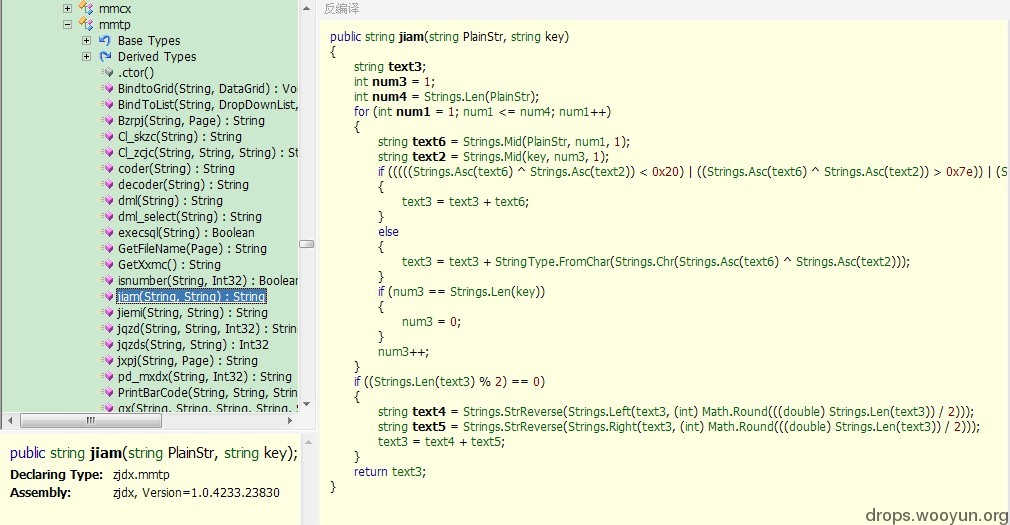## 0x01 编解码

``````a=e
b=f
c=g
……
v=z
w=a
x=b
y=c
z=d
``````

``````① 如：bcdef=fghij   cdefg=ghijk…… 这样的加密不需要工具，目测到规律然后还原。
② 略复杂一点的加密算法，密文找不到规律，可以防止用户的破解。但是如果加密解密算法侧漏了，那样所有用户的密码都会惨遭破解！
③ 复杂的加密算法，使用自定义Key加密明文，也就是说密码是明文和Key的某种运算之后生成的。这种算法有个优点，就算算法侧漏，没有Key也是无法解密的(下面开始讨论)。类似：pass=encode(str,key); str=decode(pass,key) 。
④ 更复杂的算法……(待补充)
``````

## 0x02 再议“正方”之jiam、jiemi

``````① 有多组密文，已知几组密文对应的明文和加密算法，但是由于无法获得加密所使用的Key，无法解密其它密文。
② 由系统某缺陷可以获得加密后的密文（当然明文是自己的密码这个是可控的），获得了别人的密文后由于无Key，无法解密。
````````````public string jiam(string PlainStr, string key)
{
string text3;
int num3 = 1;
int num4 = Strings.Len(PlainStr);//取密码明文长度
for (int num1 = 1; num1 <= num4; num1++)
{
string text6 = Strings.Mid(PlainStr, num1, 1);//从第一位开始每一次循环取密码中的下一个字符
string text2 = Strings.Mid(key, num3, 1);
if (((((Strings.Asc(text6) ^ Strings.Asc(text2)) < 0x20) | ((Strings.Asc(text6) ^ Strings.Asc(text2)) > 0x7e)) | (Strings.Asc(text6) < 0)) | (Strings.Asc(text6) > 0xff))//将明文中截到的某位字符的ASSCII码和Key中截到的某位字符的ASSCII码进行异或运算，若结果是不可打印字符的ASSCII码则临时字串直接加上明文中截到的这个字符
{
text3 = text3 + text6;
}
else//若异或后的结果是可打印字符的ASSCII码(33-126)，则临时字串加上ASSCII码是异或结果的字符
{
text3 = text3 + StringType.FromChar(Strings.Chr(Strings.Asc(text6) ^ Strings.Asc(text2)));
}
if (num3 == Strings.Len(key))//假如Key中截到的字符用到最后一位了，则从头开始使用Key
{
num3 = 0;
}
num3++;
}
if ((Strings.Len(text3) % 2) == 0)//假如最后的临时结果字符长度是偶数个，那么最终结果=反转字符的前半部分+反转字符的后半部分
{
string text4 = Strings.StrReverse(Strings.Left(text3, (int) Math.Round(((double) Strings.Len(text3)) / 2)));
string text5 = Strings.StrReverse(Strings.Right(text3, (int) Math.Round(((double) Strings.Len(text3)) / 2)));
text3 = text4 + text5;
}
return text3;//返回最终结果
}
``````

ASSCII码对照表请参见：http://www.96yx.com/tool/ASC2.htm 33-126为可打印字符。

``````Public Function jiam(ByVal PlainStr As String, ByVal key As String) As String
Dim strChar, KeyChar, NewStr As String
Dim Pos As Integer
Dim i, intLen As Integer
Dim Side1, Side2 As String
Pos = 1
For i = 1 To Len(PlainStr)
strChar = Mid(PlainStr, i, 1)
KeyChar = Mid(key, Pos, 1)
If ((Asc(strChar) Xor Asc(KeyChar)) < 32) Or ((Asc(strChar) Xor Asc(KeyChar)) > 126) Or (Asc(strChar) < 0) Or (Asc(strChar) > 255) Then
NewStr = NewStr & strChar
Else
NewStr = NewStr & Chr(Asc(strChar) Xor Asc(KeyChar))
End If
If Pos = Len(key) Then Pos = 0
Pos = Pos + 1
Next
If Len(NewStr) Mod 2 = 0 Then
Side1 = StrReverse(Left(NewStr, CInt((Len(NewStr) / 2))))
Side2 = StrReverse(Right(NewStr, CInt((Len(NewStr) / 2))))
NewStr = Side1 & Side2
End If
jiam = NewStr
End Function
``````

``````public string jiemi(string PlainStr, string key)
{
string text3;
int num2 = 1;
if ((Strings.Len(PlainStr) % 2) == 0)
{
string text4 = Strings.StrReverse(Strings.Left(PlainStr, (int) Math.Round(((double) Strings.Len(PlainStr)) / 2)));
string text5 = Strings.StrReverse(Strings.Right(PlainStr, (int) Math.Round(((double) Strings.Len(PlainStr)) / 2)));
PlainStr = text4 + text5;
}
int num3 = Strings.Len(PlainStr);
for (int num1 = 1; num1 <= num3; num1++)
{
string text6 = Strings.Mid(PlainStr, num1, 1);
string text2 = Strings.Mid(key, num2, 1);
if (((((Strings.Asc(text6) ^ Strings.Asc(text2)) < 0x20) | ((Strings.Asc(text6) ^ Strings.Asc(text2)) > 0x7e)) | (Strings.Asc(text6) < 0)) | (Strings.Asc(text6) > 0xff))
{
text3 = text3 + text6;
}
else
{
text3 = text3 + StringType.FromChar(Strings.Chr(Strings.Asc(text6) ^ Strings.Asc(text2)));
}
if (num2 == Strings.Len(key))
{
num2 = 0;
}
num2++;
}
return text3;
}
``````

+–判断密文长度是否是偶数|下一步
|是(重组密文) 否|下一步
|一位位截得密码和key的某位异或|下一步
|根据异或结果组合字符|下一步
+最终结果|

``````Public Function jiemi(ByVal PlainStr As String, ByVal key As String) As String
Dim strChar, KeyChar, NewStr As String
Dim Pos As Integer
Dim i As Integer
Dim Side1 As String
Dim Side2 As String
Pos = 1
If Len(PlainStr) Mod 2 = 0 Then
Side1 = StrReverse(Left(PlainStr, CInt((Len(PlainStr) / 2)))) '反转明文密码最左边一半的字符
Side2 = StrReverse(Right(PlainStr, CInt((Len(PlainStr) / 2)))) '反转明文密码最右边一半的字符
PlainStr = Side1 & Side2
End If
For i = 1 To Len(PlainStr)
strChar = Mid(PlainStr, i, 1) '一个一个处理plainstr中的字符
KeyChar = Mid(key, Pos, 1) '循环使用key中的字符
If ((Asc(strChar) Xor Asc(KeyChar)) < 32) Or ((Asc(strChar) Xor Asc(KeyChar)) > 126) Or (Asc(strChar) < 0) Or (Asc(strChar) > 255) Then
NewStr = NewStr & strChar
Else
NewStr = NewStr & Chr(Asc(strChar) Xor Asc(KeyChar))
End If
If Pos = Len(key) Then Pos = 0
Pos = Pos + 1
Next
jiemi = NewStr
End Function
``````

``````Public Function GetKey(ByVal PlainStr As String, ByVal Pass As String) As String
Dim strChar, KeyChar, NewStr As String
Dim Pos As Integer
Dim i As Integer
Dim Side1 As String
Dim Side2 As String
Pos = 1
If Len(PlainStr) Mod 2 = 0 Then
Side1 = StrReverse(Left(PlainStr, CInt((Len(PlainStr) / 2)))) '反转明文密码最左边一半的字符
Side2 = StrReverse(Right(PlainStr, CInt((Len(PlainStr) / 2)))) '反转明文密码最右边一半的字符
PlainStr = Side1 & Side2
End If
For i = 1 To Len(PlainStr)
strChar = Mid(PlainStr, i, 1) '一个一个处理plainstr中的字符
KeyChar = Mid(Pass, Pos, 1) '循环使用pass中的字符
If strChar = KeyChar Then
NewStr = NewStr & "*"
Else
NewStr = NewStr & Chr(Asc(strChar) Xor Asc(KeyChar))
End If
Pos = Pos + 1
Next
GetKey = NewStr
End Function
``````

``````A***l*36*j*
Ac**lf****w
A*xy*f*65*w
Acxy*f3**j*
……
``````

## 0x03 反观Base64编码

Base64的介绍请看：http://zh.wikipedia.org/zh-cn/Base64

Base64的逻辑是：字符串>>每个字符的8位二进制连接>>每6位转换为十进制对应编码表连接转换后字符；如果要编码的字节数不能被3整除，最后会多出1个或2个字节，那么可以使用下面的方法进行处理：先使用0字节值在末尾补足，使其能够被3整除，然后再进行base64的编码。在编码后的base64文本后加上一个或两个’=’号，代表补足的字节数。

Base标准编码表：A   QQ==
BC  QkM=

### Step1:明文转为8位二进制：

00110001001100010100001101000001001101000011011000110111010000110011010101000010001100010100001100110011010000110011000001000001010000100011000101000100001101100100001100111000010000010011100000110001001100010011000000110100010000110100001100111000001101100011100001000011010001000100001100110000010000010011100100110001

### Step2:每6位二进制数转为十进制(我以|分割便于查看)：

12|19|5|3|16|19|16|54|13|52|12|53|16|35|5|3|12|52|12|48|16|20|8|49|17|3|25|3|14|4|4|56|12|19|4|48|13|4|13|3|14|3|24|56|16|52|17|3|12|4|4|57|12|16|

### Step3对应加密后字串：

mtfdqtq2n0m1qJfdm0mWquiXrdzdoee4mteWnendody4q0rdmee5mq==

``````……
Bin(A)= 01000001  Base64(A)=编码表中第16位
Bin(E)= 01000101  Base64(E)=编码表中第17位
Bin(I)= 01001001  Base64(I)=编码表中第18位
Bin(M)= 01001101  Base64(M)=编码表中第19位
Bin(Q)= 01010001  Base64(Q)=编码表中第20位
Bin(U)= 01010101  Base64(U)=编码表中第21位
Bin(Y)= 01011001  Base64(Y)=编码表中第22位
……
``````

``````abcdefghijklmnopqrstuvwxyzABCDEFGHIJKLMNOPQRSTUVWXYZ0123456789+/=
``````

``````……
Base64(Base64(A))= Base64(编码表中第16位的字符)
Base64(Base64(E))= Base64(编码表中第17位的字符)
Base64(Base64(I))= Base64(编码表中第18位的字符)
……
``````

## 0x04 反思[微信] 扫描二维码打赏[支付宝] 扫描二维码打赏